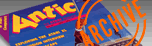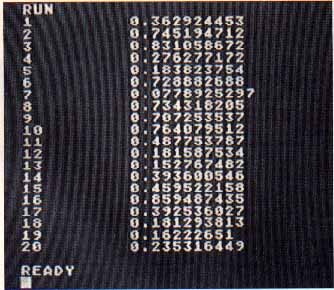`ANTIC VOL. 7, NO. 11 / MARCH 1989`

Type-In Software

# RANDOM ATARI

## Enhancing the number generator

By David McIntosh

Random Atari is a teaching article and utility program that explains a Ilttle-known technique of generating a repeatable series of "pseudo-random" numbers that make it easier for you to debug your programs. The technique also produces a much wider range of random numbers. This BASIC program works on all 8-bit Atari computers of any memory size, with disk or cassette.

The Atari 8-bit computer has excellent random number generator. In fact, its biggest drawback is that the numbers it produces are too random!

Random numbers are generated on the Atari by measuring random noise on an electronic circuit, and converting this to a value between zero and 65,535. This is divided by 65,536, to give a value between zero and one. These are excellent random numbers but they are non-repeatable. If you run a program whose results depend on random numbers, you will never be able to repeat those same results.

Being able to repeat results can be very important. For example, tracking down an intermittent bug in a non-repeatable program can be a real nightmare.

If only the error could be produced consistently, it would be much easier to find and correct. Or maybe you want to test various scenarios on your program, but can't be sure the test results were successful because you never get the same results twice. Or you have written a game with a beginner mode, and want the same obstacles repeated every time, while the advanced mode is to be random.

Most computers have a very different type of random number generator, based on a mathematical formula. An initial number, called the random seed, is taken from somewhere. If the seed is entered directly by the user, the series of psuedo-random numbers generated can be repeated, simply by using the same "random" seed. Using the repeatable numbers makes program testing and debugging much simpler.

For truly random results, the random seed must be randomly generated in some manner. On an Atari you can simply use the built-in random number function. On other computers timing is often used to generate random numbers.

Once a random seed is obtained, the numbers are generated through a simple formula. The random seed value X is multiplied by a constant value C, and then divided by another constant, M. The remainder R is a number between 1 and M-l, and is divided by M to give the the required random value between zero and one. X is then set equal to R, to seed the next random number.

The constants used in this program are:

C=397,204,094
M=2^31-1=2,147,483,647

GETTING STARTED

Type in Listing 1, RANDOM.BAS, check it with TYPO II, and SAVE a copy. If you have trouble with the special characters in lines 20090- 20090, 20120, 20140 and 20210, don't type them. Listing 2 will create them for you. Type in Listing 2, check it with TYPO II and SAVE it. When you RUN Listing 2 it creates a file called LINES.LST. Merge this file with Listing 1 by typing LOAL) "D:RANDOM.BAS and then ENTER "D:LINES. LST': (Cassette owners use ENTER "C:"). Remember to SAVE the completed program before you RUN it.

Listing 3, RANDOM.M65, is the MAC/65 source code for the program. You do not need to type it in to use Random Atari.

To RUN Random Atari, you need to set a variable X to a number between zero and M, and then enter GOSUB 20000. The following lines will set X and then call Random Atari.

100 X=12345:GOSUB 20000
110 FOR I=l TO 20:GOSUB 20200:PRINT LX:NEXT I
120 END

Here, the random seed is 12345. Every time this is RUN, the same 20 random values will be produced. Now try setting X to zero on line 100. The program will generate a truly random seed of its own, and a different 20 values will be produced every time the program is RUN.

The value of X should be set only once in your program, preferably near the beginning. If X is between one and M, then X is the random seed, and the program results can be reproduced by reusing the same value. If X is zero or less, a random seed is chosen using the regular random number generator, and the results will be unpredictable.

Random Atari gives you more than just the option of repeatable random numbers--it also gives you a higher degree of variability. The BASIC RND(0) command can produce only 65,536 distinct values. For some purposes, this is not enough. Random Atari, on the other hand, produces 2,147,483,646 distict values.PROGRAM TAKE-APART

Line 20010. The program uses three variables, X\$, X(0), and X. These are three different values, in spite of the similarity of their names. On this line, X(0) is set equal to its own address in memory, which is the byte immediately following the address of X\$, since they were DIMensioned consecutively.

Line 20020. POKEs the address of X(0) into locations 203 and 204 (\$CB and \$CC). These are two Page Zero memory locations which BASIC doesn't use.

Line 20060. If X (the random seed) is zero or negative, calculate a new random seed. This will be a value between one and 2^31-1.

Line 20065. If X is greater than 2^31-2, then set X to equal 2^31-2.

Line 20070. Convert the random seed to a four-byte binary number, and store it in memory locations 205-208 (\$CD--\$D0).

Line 20080. X(0) is set to the address of a machine language routine which copies a section of memory to a new location.

Lines 20090-20150. The machine lanuage random number generator is copied to Page Six in memory locations 1536-1785 (\$0600-\$06F9).

Line 20160. Return from the initialization routine.

Lines 20200-20210. Call this routine every time you need a random number. These lines call a machine language subroutine which calculates a "pseudo-random" number betareen zero and one, then places this value in X(0). The variable X is then assigned this value.

ASSEMBLER TAKE-APART

line 40. The program is compiled at location 25600 (\$6400), but everything down to line 280 is fully relocatable.

Lines 50-130. Store the value 337,204,094 as a binary integer in locations 219-222. These locations are usually referred to as FRE.

Lines 140-180. Move the random seed (or the remainder from the last calculation) from locations 205-208 to locations 213-216 (FR0).

Line 190. Multiply the random seed in FRO by the constant value in FRE. The result is stored in locations 225-232, FR1. Note that the result of multiplying two four-byte values is an eight-byte value.

Line 200. Calculate the remainder when FR1 is divided by 2^31-1. The four-byte result is contained in bytes 229-232 of FR1.

Lines 210-250. Replace the random seed at locations 205-208 with the remainder calculated above for the next calculation.

Lines 260-270. Convert the calculated value from a binary integer to a floating point decimal value between 0 and 1. The value is first doubled by the ROTATL routine, because the routine BTOD assumes we have a value between 1 and 2^32-1, where to this point we have calculated a value between 1 and 2^31-1.

This routine divides the four-byte binary integer at FR1 by 2^31. The random number algorithm actually requires FR1 to be divided by 2^31-1, but the difference is negligible.

.Line 290. The remainder of this program is compiled starting at location 1536 (\$0600) and is not relocatable.

Lines 300-580. Routine to perform binary integer multiplication. Two four-byte integers at FR0 and FRE are multiplied, and the resulting eight-byte value is stored in FR1.

Lines 590-670. Add the contents of FR0 to FR1.

Lines 680-1010. Calculate the remainder when the value at FR1 is divided by 2^31-1. A little algebra here saved me from writing a complicate binary division routine.

Let A % B represent the remainder when A is divided by B (as it does in some versions of BASIC), and let X=M*2^31+N, where N<2^31. Then we can say:

```X%(2^31-1)=(M*2^31+N)%(2^31-1)
=(M+N)%(2^31-1)
```

What started as a complicated division problem is now a simple addition problem. The routine repeatedly adds the top 33 bits of FR1 to the bottom 31 bits of FR1 (M and N from above), until the top 33 bits are all zeroes. The bottom 31 bits contain the required remainder.

Lines 1020-1450. Convert a four-byte binary fraction at FR1 to a floating point decimal number. The result is stored at the six-byte location starting at the byte pointed to by locations 203 and 204. The binary fraction is assumed to be positive, and its 32 bits are assumed to represent halves, quarters, eighths, sixteenths, etc.

The routine repeatedly multiplies the fraction by 10 until it calculates a value greater than 1. Every other time it is multiplied, the exponent of the floating point value is decremented, having started from an initial value of 63.

Once the value exceeds 1, the non-fractional part of the number becomes the first digit of the floating point value. The non-fractional part is set to zero, and the fraction is again multiplied by 10 to get the next digit of the floating point value. This is repeated until the five bytes of the floating point value each contain two digits.

Lines 1460-1520. Copy four bytes from FRI to FR0.

Lines 1530-1630. Binary multiplication of FR0 by 10. The result is stored in five bytes of FR1. Before. executing this routine, it is assumed that FR1 has been set equal to FR0.

Lines 1640-1700. Rotate five bytes of FR1 one bit to the left. This is equivalent to multiplying FR1 by two.

REFERENCE

"An introduction to Stochastic Simulation," Chicago: Society of Actuaries, #130-033-86.

David McIntosh is an actuary and programmer for National Life of Canada.